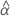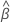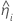# Methods and formulas for the shelf life for Stability Study for random batches

Select the method or formula of your choice.

## Shelf life for a model with a random batch factor and only a lower specification limit

To simplify the calculation of the shelf life, consider whether the model includes the time*batch interaction or not.

### The model with time, batch, and the time*batch interaction

When the batch effect and the batch*time interaction are in the model, the fit for the ith batch at time xij uses this model:

where
TermDescriptionthe estimated fixed mean intercept for all batchesthe estimated mean slope for all batchesthe estimated random intercept for the ith batchthe estimated slope for the ith batch
For this model, the (1 - p)th percentile of the response is:
We assume that these equalities are true:
The variance of the estimated percentile is:
where
To calculate a meaningful shelf life, Minitab evaluates two conditions. First, Minitab determines whether the estimated fixed mean intercept for all batches is positive:
Second, Minitab determines whether the estimated mean slope for all batches is negative:
To find the shelf life, set the equation that follows equal to the lower specification limit and solve for time (x):

Minitab uses an iterative algorithm to find a solution between 0 and 10 times the largest value in the time variable.

### The model with time and batch

When the batch*time interaction is not in the model, the slopes are the same for every batch. The fit for the ith batch at time xij uses this model:
where
TermDescriptionthe estimated fixed mean intercept for all batchesthe estimated mean slope for all batchesthe estimated random intercept for the ith batch
For this model, the (1 - p)th percentile of the response is:
We assume that these equalities are true:
The variance of the estimated percentile is:
where
Substituting these values into the formula for the variation of the percentile yields:
where
To calculate a meaningful shelf life, Minitab evaluates three conditions. First, Minitab determines whether the estimated fixed mean intercept for all batches is positive:
Second, Minitab determines whether the estimated mean slope for all batches is negative:
Third, Minitab determines whether the square root portion of the quadratic equation has a real number solution:
where
To find the shelf life, set the equation that follows equal to the lower specification limit and solve for time (x):
For this model, the solution for x simplifies to this formula:

### Notation

TermDescriptionthe estimated fixed mean intercept for all batchesthe estimated mean slope for all batches
Zthe value of the inverse cumulative distribution function
Lthe lower specification limit
xthe shelf life
pthe proportion of the product above the lower specification limit
Xthe design matrix

## Shelf life for a model with a random batch factor and only an upper specification limit

To simplify the calculation of the shelf life, consider whether the model includes the time*batch interaction or not.

### The model with time, batch, and the time*batch interaction

When the batch effect and the batch*time interaction are in the model, the fit for the ith batch at time xij uses this model:

where
TermDescriptionthe estimated fixed mean intercept for all batchesthe estimated mean slope for all batchesthe estimated random intercept for the ith batchthe estimated slope for the ith batch
For this model, the pth percentile of the response is:
We assume that these equalities are true:
The variance of the estimated percentile is:
where
To calculate a meaningful shelf life, Minitab evaluates two conditions. First, Minitab determines whether the estimated fixed mean intercept for all batches is positive:
Second, Minitab determines whether the estimated mean slope for all batches is negative:
To find the shelf life, set the equation that follows equal to the lower specification limit and solve for time (x):

Minitab uses an iterative algorithm to find a solution between 0 and 10 times the largest value in the time variable.

### The model with time and batch

When the batch*time interaction is not in the model, the slopes are the same for every batch. The fit for the ith batch at time xij uses this model:
where
TermDescriptionthe estimated fixed mean intercept for all batchesthe estimated mean slope for all batchesthe estimated random intercept for the ith batch
For this model, the pth percentile of the response is:
We assume that these equalities are true:
The variance of the estimated percentile is:
where
Substituting these values into the formula for the variation of the percentile yields:
where
To calculate a meaningful shelf life, Minitab evaluates three conditions. First, Minitab determines whether the estimated fixed mean intercept for all batches is positive:
Second, Minitab determines whether the estimated mean slope for all batches is negative:
Third, Minitab determines whether the square root portion of the quadratic equation has a real number solution:
where
To find the shelf life, set the equation that follows equal to the lower specification limit and solve for time (x):
For this model, the solution for x simplifies to this formula:

### Notation

TermDescriptionthe estimated fixed mean intercept for all batchesthe estimated mean slope for all batches
Zthe value of the inverse cumulative distribution function
Lthe lower specification limit
xthe shelf life
pthe proportion of the product above the lower specification limit
Xthe design matrix

## Both limits

Minitab first determines whether a meaningful estimate of the shelf life exists. A meaningful estimate of the shelf life exists when the mean response is between the upper and lower specification limits at time 0:

If a meaningful estimate exists, then Minitab determines whether to estimate the shelf life relative to the upper specification limit or the lower specification limit.

If the response decreases with time, then Minitab calculates the shelf life relative to the lower specification limit. This formula gives the condition when the response decreases:
If the response increases with time, then Minitab calculates the shelf life relative to the upper specification limit. This formula gives the condition when the response increases:

For details on the calculation of the shelf life for each case, go to the section that describes the calculation when there is only one specification limit. To calculate the shelf life when the calculations have two limits that are both relevant to the shelf life, change Zcl and Z0.95 to Z0.5+cl/2.

### Notation

TermDescriptionthe estimated fixed mean intercept for all batchesthe estimated mean slope for all batches
clthe confidence level
By using this site you agree to the use of cookies for analytics and personalized content.  Read our policy